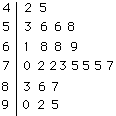Math Central - mathcentral.uregina.caResource Room— glossaries Resource RoomMathematics Glossary - Middle Years Maxine Stinka, Saskatchewan Education, Curriculum and Instruction Note: The definitions included here are those that are used in the Saskatchewan Education document "Mathematics 6-9: A Curriculum Guide for the Middle Level". Various mathematics dictionaries may have different definitions. These definitions are designed to be meaningful to middle level mathematics teachers. Select the first letter of the term that you wish to look for. A | B | C | D | E | F | H | I | J | K | L | M | N | O | P | Q | R | S | T | U | V | W | X | Y | Z S sample refers to a representative portion of the population from which information is gathered. Often, the information is used to draw conclusions about the behavior of the population. e.g. To determine the favourite activity of students in your school, you could collect the information from some of the students (a sample). The sample should be random and representative of the group. scale factor the ratio of a distance measured on a scale drawing to the corresponding distance measured on the actual object. scalene triangle a triangle with all sides of different lengths. scatterplot a graphical method used in statistics to show the relationship between two variables. The values of the two variables form a ordered pairs that are graphed on the coordinate plane. scientific notation writing a number as the product of a number between 1 and 10 and the appropriate power of ten. e.g. 118 000 = 1.18 X 105. secant of circle a line that intersects a circle in two points. secondary data data obtained indirectly from sources such as a book or computer database. similar polygons - polygons that have the same shape but not necessarily the same size. simple interest see interest simplest form (lowest terms) a fraction is in simplest form if both its numerator and denominator are whole numbers and their only common factor is 1. simplified fraction a fraction in simplest form. spreadsheet a computer generated arrangement of data in rows and columns. square root a number that when squared gives the value of the original number. e.g. The square root of 25 = 5 because 52 = 25. straight angle an angle whose measure is 180o. stem-and-leaf plot in statistics, a way of recording, organizing and displaying numerical data so that the original data remains intact. e.g.In this plot, the last row represents the numbers 90, 92 and 95. supplementary angles two angles whose measures total 180o. surface area the sum of the areas of all the faces, including the bases, of a 3-D object. Questions or comments about the glossary can be directed to TheCentralizer@MathCentral.uregina.ca or see About Us page for more information.
 Math Central is supported by the University of Regina and the Imperial Oil Foundation.about math central :: site map :: links :: notre site français* Registered trade mark of Imperial Oil Limited. Used under license.